�� �� �� ��
����ID:
����ԭ��: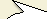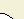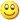To: ��������ʦ���ã�Ϊʲô�ڲ�ѯ��ʱ���һ��fetch�� cr=3,r=1?ÿ�β�ѯ��ʱ���һ��fetch�ģ�r = 1 ? SELECT * FROM EMP END OF STMT PARSE #1:c=0,e=435,p=0,cr=0,cu=0,mis=1,r=0,dep=0,og=4,tim=966751342 EXEC #1:c=0,e=35,p=0,cr=0,cu=0,mis=0,r=0,dep=0,og=4,tim=966753976 FETCH #1:c=0,e=47,p=0,cr=3,cu=0,mis=0,r=1,dep=0,og=4,tim=966754543 FETCH #1:c=0,e=66,p=0,cr=1,cu=0,mis=0,r=13,dep=0,og=4,tim=966755534 From: gao2012.06.03 17:47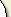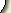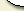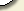��������: �������������, �����޷��ظ�.
�����ǳ�:
��Ϣͼ��: ( ��� )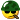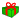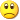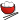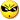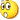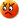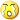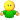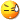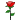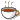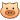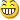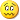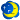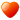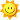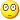BB ����:
����:

�����ɾ���ظ�, �����������ɾ���ظ���ť.
 ɾ �� �� �� ��������: �������������, ֻ�а�������ɾ���ظ�.